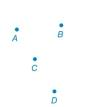Chapter 1.3, Problem 10E### Elementary Geometry for College St...

6th Edition
Daniel C. Alexander + 1 other
ISBN: 9781285195698

#### Solutions

Chapter
Section### Elementary Geometry for College St...

6th Edition
Daniel C. Alexander + 1 other
ISBN: 9781285195698
Textbook Problem
28 views

# In Exercises 9 to 28, use the drawings as needed to answer the following questions.How many lines can be drawn througha) point A?b) points A and B?c) points A, B, and C?d) points A, B, and D?Exercises 9, 10

To determine

a)

To find:

The number of lines drawn through the given points.

Explanation

Given:

The given figure is,

The given point to draw number of line is point A.

Approach:

Consider the figure with lines from A.

To determine

b)

To find:

The number of lines drawn through the given points.

To determine

c)

To find:

The number of lines drawn through the given points.

To determine

d)

To find:

The number of lines drawn through the given points.

### Still sussing out bartleby?

Check out a sample textbook solution.

See a sample solution

#### The Solution to Your Study Problems

Bartleby provides explanations to thousands of textbook problems written by our experts, many with advanced degrees!

Get Started

#### Evaluate the integral. 0141+p2dp

Single Variable Calculus: Early Transcendentals, Volume I

#### In Exercises 29-34, rationalize the denominator of each expression. 31. 1xy

Applied Calculus for the Managerial, Life, and Social Sciences: A Brief Approach

#### True or False: x2+1x3dx may be solved using a trigonometric substitution.

Study Guide for Stewart's Single Variable Calculus: Early Transcendentals, 8th